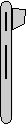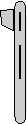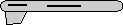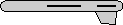# Maths - Euler to Axis-Angle - Sample Orientations

## Sample Rotations

In order to try to explain things and give some examples we can try I thought it might help to show the rotations for a finite subset of the rotation group. We will use the set of rotations of a cube onto itself, this is a permutation group which gives 24 possible rotations as explaned on this page.

heading applied first giving 4 possible orientations:reference orientation heading = 0 attitude = 0 bank = 0 angle = 2 * acos(c1c2c3 - s1s2s3) x = s1 s2 c3 +c1 c2 s3 y = s1 c2 c3 + c1 s2 s3 z = c1 s2 c3 - s1 c2 s3 where: c1 = cos(heading/2) =1 c2 = cos(attitude/2) =1 c3 = cos(bank/2) =1 s1 = sin(heading/2) =0 s2 = sin(attitude/2) =0 s3 = sin(bank/2) =0 which gives: angle = 0 x = 0 y = 0 z = 0 Normalise axis, since angle is 0 axis can be anything so set axis to (1,0,0) angle = 0 degrees axis = 1,0,0rotate by 90 degrees about y axis heading = 90 degrees attitude = 0 bank = 0 angle = 2 * acos(c1c2c3 - s1s2s3) x = s1 s2 c3 +c1 c2 s3 y = s1 c2 c3 + c1 s2 s3 z = c1 s2 c3 - s1 c2 s3 where: c1 = cos(heading/2) =0.7071 c2 = cos(attitude/2) =1 c3 = cos(bank/2) =1 s1 = sin(heading/2) =0.7071 s2 = sin(attitude/2) =0 s3 = sin(bank/2) =0 which gives: angle = 90 degrees x = 0 y = 0.7071 z = 0 Normalising axis gives: angle = 90 degrees axis = 0,1,0rotate by 180 degrees about y axis heading = 180 degrees attitude = 0 bank = 0 angle = 2 * acos(c1c2c3 - s1s2s3) x = s1 s2 c3 +c1 c2 s3 y = s1 c2 c3 + c1 s2 s3 z = c1 s2 c3 - s1 c2 s3 where: c1 = cos(heading/2) =0 c2 = cos(attitude/2) =1 c3 = cos(bank/2) =1 s1 = sin(heading/2) =1 s2 = sin(attitude/2) =0 s3 = sin(bank/2) =0 which gives: angle = 180 degrees x = 0 y = 1 z = 0 axis is already normalised angle = 180 degrees axis = 0,1,0rotate by 270 degrees about y axis heading = -90 degrees attitude = 0 bank = 0 angle = 2 * acos(c1c2c3 - s1s2s3) x = s1 s2 c3 +c1 c2 s3 y = s1 c2 c3 + c1 s2 s3 z = c1 s2 c3 - s1 c2 s3 where: c1 = cos(heading/2) =0.7071 c2 = cos(attitude/2) =1 c3 = cos(bank/2) =1 s1 = sin(heading/2) =-0.7071 s2 = sin(attitude/2) =0 s3 = sin(bank/2) =0 which gives: angle = 90 degrees x = 0 y = -0.7071 z = 0 Normalising axis gives: angle = 90 degrees axis = 0,-1,0 or angle = -90 degrees axis = 0,1,0

Then apply attitude +90 degrees for each of the above: (note: that if we went on to apply bank to these it would just rotate between these values, the straight up and streight down orientations are known as singularities because they can be fully defined without using the bank value) post multiply above by 0.7071 + k 0.7071 to give:heading = 0 attitude = 90 degrees bank = 0 angle = 2 * acos(c1c2c3 - s1s2s3) x = s1 s2 c3 +c1 c2 s3 y = s1 c2 c3 + c1 s2 s3 z = c1 s2 c3 - s1 c2 s3 where: c1 = cos(heading/2) =1 c2 = cos(attitude/2) =0.7071 c3 = cos(bank/2) =1 s1 = sin(heading/2) =0 s2 = sin(attitude/2) =0.7071 s3 = sin(bank/2) =0 which gives: angle = 90 degrees x = 0 y = 0 z = 0.7071 Normalising axis gives: angle = 90 degrees axis = 0,0,1heading = 90 degrees attitude = 90 degrees bank = 0 angle = 2 * acos(c1c2c3 - s1s2s3) x = s1 s2 c3 +c1 c2 s3 y = s1 c2 c3 + c1 s2 s3 z = c1 s2 c3 - s1 c2 s3 where: c1 = cos(heading/2) =0.7071 c2 = cos(attitude/2) =0.7071 c3 = cos(bank/2) =1 s1 = sin(heading/2) =0.7071 s2 = sin(attitude/2) =0.7071 s3 = sin(bank/2) =0 which gives: angle = 120 degrees x = 0.5 y = 0.5 z = 0.5 Normalising axis gives: angle = 120 degrees axis = 0.5774,0.5774,0.5774heading = 180 degrees attitude = 90 degrees bank = 0 angle = 2 * acos(c1c2c3 - s1s2s3) x = s1 s2 c3 +c1 c2 s3 y = s1 c2 c3 + c1 s2 s3 z = c1 s2 c3 - s1 c2 s3 where: c1 = cos(heading/2) =0 c2 = cos(attitude/2) =0.7071 c3 = cos(bank/2) =1 s1 = sin(heading/2) =1 s2 = sin(attitude/2) =0.7071 s3 = sin(bank/2) =0 which gives: angle = 180 degrees x = 0.7071 y = 0.7071 z = 0 axis is already normalised: angle = 180 degrees axis = 0.7071,0.7071,0heading = -90 degrees attitude = 90 degrees bank = 0 angle = 2 * acos(c1c2c3 - s1s2s3) x = s1 s2 c3 +c1 c2 s3 y = s1 c2 c3 + c1 s2 s3 z = c1 s2 c3 - s1 c2 s3 where: c1 = cos(heading/2) =0.7071 c2 = cos(attitude/2) =0.7071 c3 = cos(bank/2) =1 s1 = sin(heading/2) =-0.7071 s2 = sin(attitude/2) =0.7071 s3 = sin(bank/2) =0 which gives: angle = 120 degrees x = -0.5 y = -0.5 z = 0.5 Normalising axis gives: angle = 120 degrees axis = -0.5774,-0.5774,0.5774

Or instead apply attitude -90 degrees (also a singularity): post multiply top row by 0.7071 - k 0.7071 to give:heading = 0 attitude = -90 degrees bank = 0 angle = 2 * acos(c1c2c3 - s1s2s3) x = s1 s2 c3 +c1 c2 s3 y = s1 c2 c3 + c1 s2 s3 z = c1 s2 c3 - s1 c2 s3 where: c1 = cos(heading/2) =1 c2 = cos(attitude/2) =0.7071 c3 = cos(bank/2) =1 s1 = sin(heading/2) =0 s2 = sin(attitude/2) =-0.7071 s3 = sin(bank/2) =0 which gives: angle = 90 degrees x = 0 y = 0 z = -0.7071 Normalising axis gives: angle = 90 degrees axis = 0,0,-1 (equivilant rotation to: angle = -90 degrees axis = 0,0,1)heading = 90 degrees attitude = -90 degrees bank = 0 angle = 2 * acos(c1c2c3 - s1s2s3) x = s1 s2 c3 +c1 c2 s3 y = s1 c2 c3 + c1 s2 s3 z = c1 s2 c3 - s1 c2 s3 where: c1 = cos(heading/2) =0.7071 c2 = cos(attitude/2) =0.7071 c3 = cos(bank/2) =1 s1 = sin(heading/2) =0.7071 s2 = sin(attitude/2) =-0.7071 s3 = sin(bank/2) =0 which gives: angle = 120 degrees x = -0.5 y = 0.5 z = -0.5 Normalising axis gives: angle = 120 degrees axis = -0.5774,0.5774,-0.5774heading = 180 degrees attitude = -90 degrees bank = 0 angle = 2 * acos(c1c2c3 - s1s2s3) x = s1 s2 c3 +c1 c2 s3 y = s1 c2 c3 + c1 s2 s3 z = c1 s2 c3 - s1 c2 s3 where: c1 = cos(heading/2) =0 c2 = cos(attitude/2) =0.7071 c3 = cos(bank/2) =1 s1 = sin(heading/2) =1 s2 = sin(attitude/2) =-0.7071 s3 = sin(bank/2) =0 which gives: angle = 180 degrees x = -0.7071 y = 0.7071 z = 0 axis is already normalised: angle = 180 degrees axis = -0.7071,0.7071,0heading = -90 degrees attitude = -90 degrees bank = 0 angle = 2 * acos(c1c2c3 - s1s2s3) x = s1 s2 c3 +c1 c2 s3 y = s1 c2 c3 + c1 s2 s3 z = c1 s2 c3 - s1 c2 s3 where: c1 = cos(heading/2) =0.7071 c2 = cos(attitude/2) =0.7071 c3 = cos(bank/2) =1 s1 = sin(heading/2) =-0.7071 s2 = sin(attitude/2) =-0.7071 s3 = sin(bank/2) =0 which gives: angle = 120 degrees x = 0.5 y = -0.5 z = -0.5 Normalising axis gives: angle = 120 degrees axis = 0.5774,-0.5774,-0.5774

Normally we dont go beond attitude + or - 90 degrees because thes are singularities, instead apply bank +90 degrees: post multiply top row by 0.7071 + i 0.7071 to give:heading = 0 attitude = 0 bank = 90 degrees angle = 2 * acos(c1c2c3 - s1s2s3) x = s1 s2 c3 +c1 c2 s3 y = s1 c2 c3 + c1 s2 s3 z = c1 s2 c3 - s1 c2 s3 where: c1 = cos(heading/2) =1 c2 = cos(attitude/2) =1 c3 = cos(bank/2) =0.7071 s1 = sin(heading/2) =0 s2 = sin(attitude/2) =0 s3 = sin(bank/2) =0.7071 which gives: angle = 90 degrees x = 0.7071 y = 0 z = 0 Normalising axis gives: angle = 90 degrees axis = 1,0,0heading = 90 degrees attitude = 0 bank = 90 degrees angle = 2 * acos(c1c2c3 - s1s2s3) x = s1 s2 c3 +c1 c2 s3 y = s1 c2 c3 + c1 s2 s3 z = c1 s2 c3 - s1 c2 s3 where: c1 = cos(heading/2) =0.7071 c2 = cos(attitude/2) =1 c3 = cos(bank/2) =0.7071 s1 = sin(heading/2) =0.7071 s2 = sin(attitude/2) =0 s3 = sin(bank/2) =0.7071 which gives: angle = 120 degrees x = 0.5 y = 0.5 z = -0.5 Normalising axis gives: angle = 120 degrees axis = 0.5774,0.5774,-0.5774heading = 180 degrees attitude = 0 bank = 90 degrees angle = 2 * acos(c1c2c3 - s1s2s3) x = s1 s2 c3 +c1 c2 s3 y = s1 c2 c3 + c1 s2 s3 z = c1 s2 c3 - s1 c2 s3 where: c1 = cos(heading/2) =0 c2 = cos(attitude/2) =1 c3 = cos(bank/2) =0.7071 s1 = sin(heading/2) =1 s2 = sin(attitude/2) =0 s3 = sin(bank/2) =0.7071 which gives: angle = 180 degrees x = 0 y = 0.7071 z = -0.7071 axis is already normalised: angle = 180 degrees axis = 0,0.7071,-0.7071heading = -90 degrees attitude = 0 bank = 90 degrees angle = 2 * acos(c1c2c3 - s1s2s3) x = s1 s2 c3 +c1 c2 s3 y = s1 c2 c3 + c1 s2 s3 z = c1 s2 c3 - s1 c2 s3 where: c1 = cos(heading/2) =0.7071 c2 = cos(attitude/2) =1 c3 = cos(bank/2) =0.7071 s1 = sin(heading/2) =-0.7071 s2 = sin(attitude/2) =0 s3 = sin(bank/2) =0.7071 which gives: angle = 120 degrees x = 0.5 y = -0.5 z = 0.5 Normalising axis gives: angle = 120 degrees axis = 0.5774,-0.5774,0.5774

Apply bank +180 degrees: post multiply top row by i to give:heading = 0 attitude = 0 bank = 180 degrees angle = 2 * acos(c1c2c3 - s1s2s3) x = s1 s2 c3 +c1 c2 s3 y = s1 c2 c3 + c1 s2 s3 z = c1 s2 c3 - s1 c2 s3 where: c1 = cos(heading/2) =1 c2 = cos(attitude/2) =1 c3 = cos(bank/2) =0 s1 = sin(heading/2) =0 s2 = sin(attitude/2) =0 s3 = sin(bank/2) =1 which gives: angle = 180 degrees x = 1 y = 0 z = 0 axis is already normalised: angle = 180 degrees axis = 1,0,0heading = 90 degrees attitude = 0 bank = 180 degrees angle = 2 * acos(c1c2c3 - s1s2s3) x = s1 s2 c3 +c1 c2 s3 y = s1 c2 c3 + c1 s2 s3 z = c1 s2 c3 - s1 c2 s3 where: c1 = cos(heading/2) =0.7071 c2 = cos(attitude/2) =1 c3 = cos(bank/2) =0 s1 = sin(heading/2) =0.7071 s2 = sin(attitude/2) =0 s3 = sin(bank/2) =1 which gives: angle = 180 degrees x = 0.7071 y = 0 z = -0.7071 axis is already normalised: angle = 180 degrees axis = 0.7071,0,-0.7071heading = 180 degrees attitude = 0 bank = 180 degrees angle = 2 * acos(c1c2c3 - s1s2s3) x = s1 s2 c3 +c1 c2 s3 y = s1 c2 c3 + c1 s2 s3 z = c1 s2 c3 - s1 c2 s3 where: c1 = cos(heading/2) =0 c2 = cos(attitude/2) =1 c3 = cos(bank/2) =0 s1 = sin(heading/2) =1 s2 = sin(attitude/2) =0 s3 = sin(bank/2) =1 which gives: angle = 180 degrees x = 0 y = 0 z = 1 axis is already normalised: angle = 180 degrees axis = 0,0,1heading = -90 degrees attitude = 0 bank = 180 degrees angle = 2 * acos(c1c2c3 - s1s2s3) x = s1 s2 c3 +c1 c2 s3 y = s1 c2 c3 + c1 s2 s3 z = c1 s2 c3 - s1 c2 s3 where: c1 = cos(heading/2) =0.7071 c2 = cos(attitude/2) =1 c3 = cos(bank/2) =0 s1 = sin(heading/2) =-0.7071 s2 = sin(attitude/2) =0 s3 = sin(bank/2) =1 which gives: angle = 180 degrees x = 0.7071 y = 0 z = 0.7071 axis is already normalised: angle = 180 degrees axis = 0.7071,0,0.7071

Apply bank -90 degrees: post multiply top row by 0.7071 - i 0.7071 to give:heading = 0 attitude = 0 bank = -90 degrees angle = 2 * acos(c1c2c3 - s1s2s3) x = s1 s2 c3 +c1 c2 s3 y = s1 c2 c3 + c1 s2 s3 z = c1 s2 c3 - s1 c2 s3 where: c1 = cos(heading/2) =1 c2 = cos(attitude/2) =1 c3 = cos(bank/2) =0.7071 s1 = sin(heading/2) =0 s2 = sin(attitude/2) =0 s3 = sin(bank/2) =-0.7071 which gives: angle = 90 degrees x = -0.7071 y = 0 z = 0 Normalising axis gives: angle = 90 degrees axis = -1,0,0 (equivilant rotation to: angle = -90 degrees axis = 1,0,0)heading = 90 degrees attitude = 0 bank = -90 degrees angle = 2 * acos(c1c2c3 - s1s2s3) x = s1 s2 c3 +c1 c2 s3 y = s1 c2 c3 + c1 s2 s3 z = c1 s2 c3 - s1 c2 s3 where: c1 = cos(heading/2) =0.7071 c2 = cos(attitude/2) =1 c3 = cos(bank/2) =0.7071 s1 = sin(heading/2) =0.7071 s2 = sin(attitude/2) =0 s3 = sin(bank/2) =-0.7071 which gives: angle = 120 degrees x = -0.5 y = 0.5 z = 0.5 Normalising axis gives: angle = 120 degrees axis = -0.5774,0.5774,0.5774heading = 180 degrees attitude = 0 bank = -90 degrees angle = 2 * acos(c1c2c3 - s1s2s3) x = s1 s2 c3 +c1 c2 s3 y = s1 c2 c3 + c1 s2 s3 z = c1 s2 c3 - s1 c2 s3 where: c1 = cos(heading/2) =0 c2 = cos(attitude/2) =1 c3 = cos(bank/2) =0.7071 s1 = sin(heading/2) =1 s2 = sin(attitude/2) =0 s3 = sin(bank/2) =-0.7071 which gives: angle = 180 degrees x = 0 y = 0.7071 z = 0.7071 axis is already normalised: angle = 180 degrees axis = 0,0.7071,0.7071heading = -90 degrees attitude = 0 bank = -90 degrees angle = 2 * acos(c1c2c3 - s1s2s3) x = s1 s2 c3 +c1 c2 s3 y = s1 c2 c3 + c1 s2 s3 z = c1 s2 c3 - s1 c2 s3 where: c1 = cos(heading/2) =0.7071 c2 = cos(attitude/2) =1 c3 = cos(bank/2) =0.7071 s1 = sin(heading/2) =-0.7071 s2 = sin(attitude/2) =0 s3 = sin(bank/2) =-0.7071 which gives: angle = 120 degrees x = -0.5 y = -0.5 z = -0.5 Normalising axis gives: angle = 120 degrees axis = -0.5774,-0.5774,-0.5774